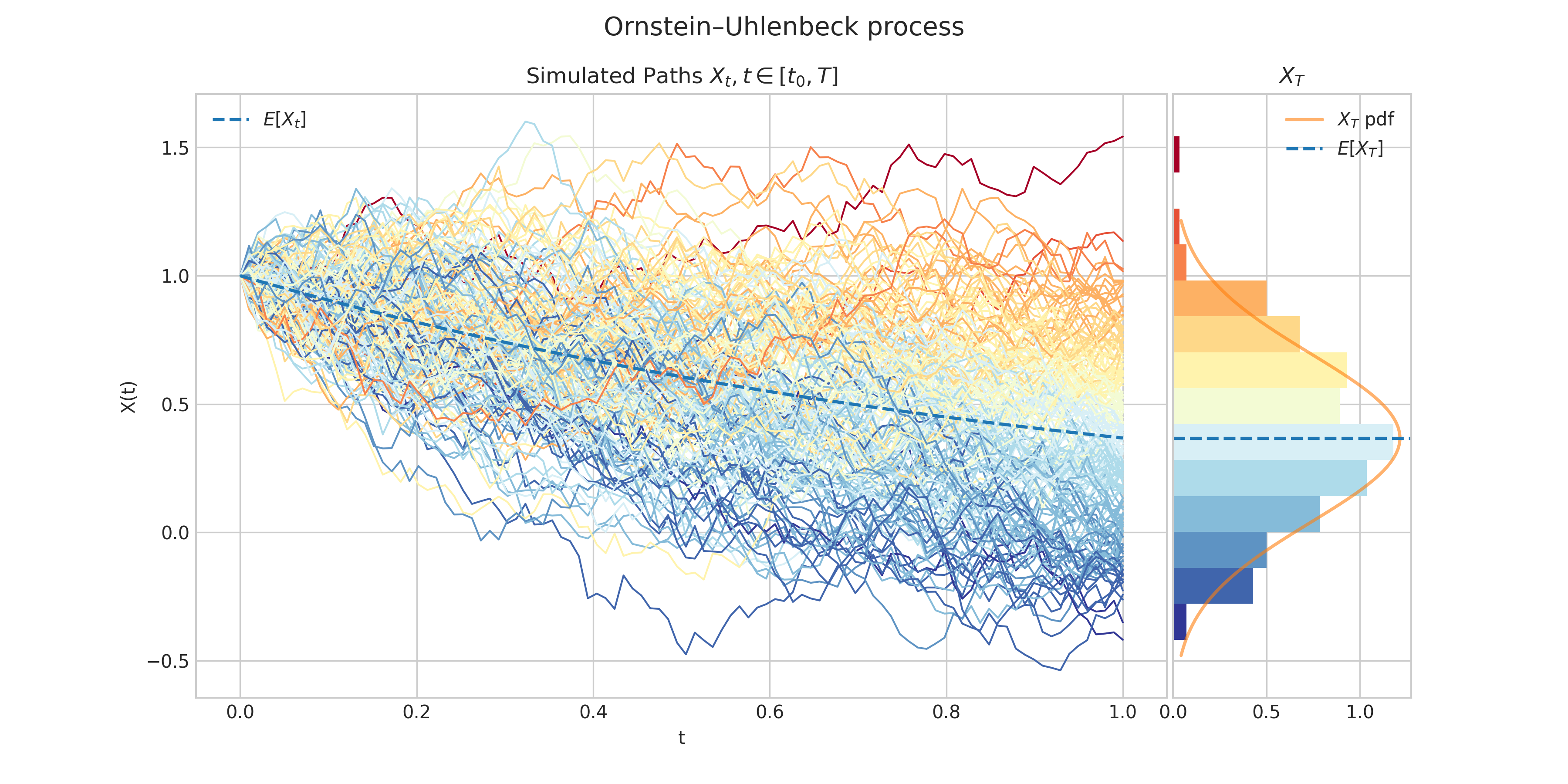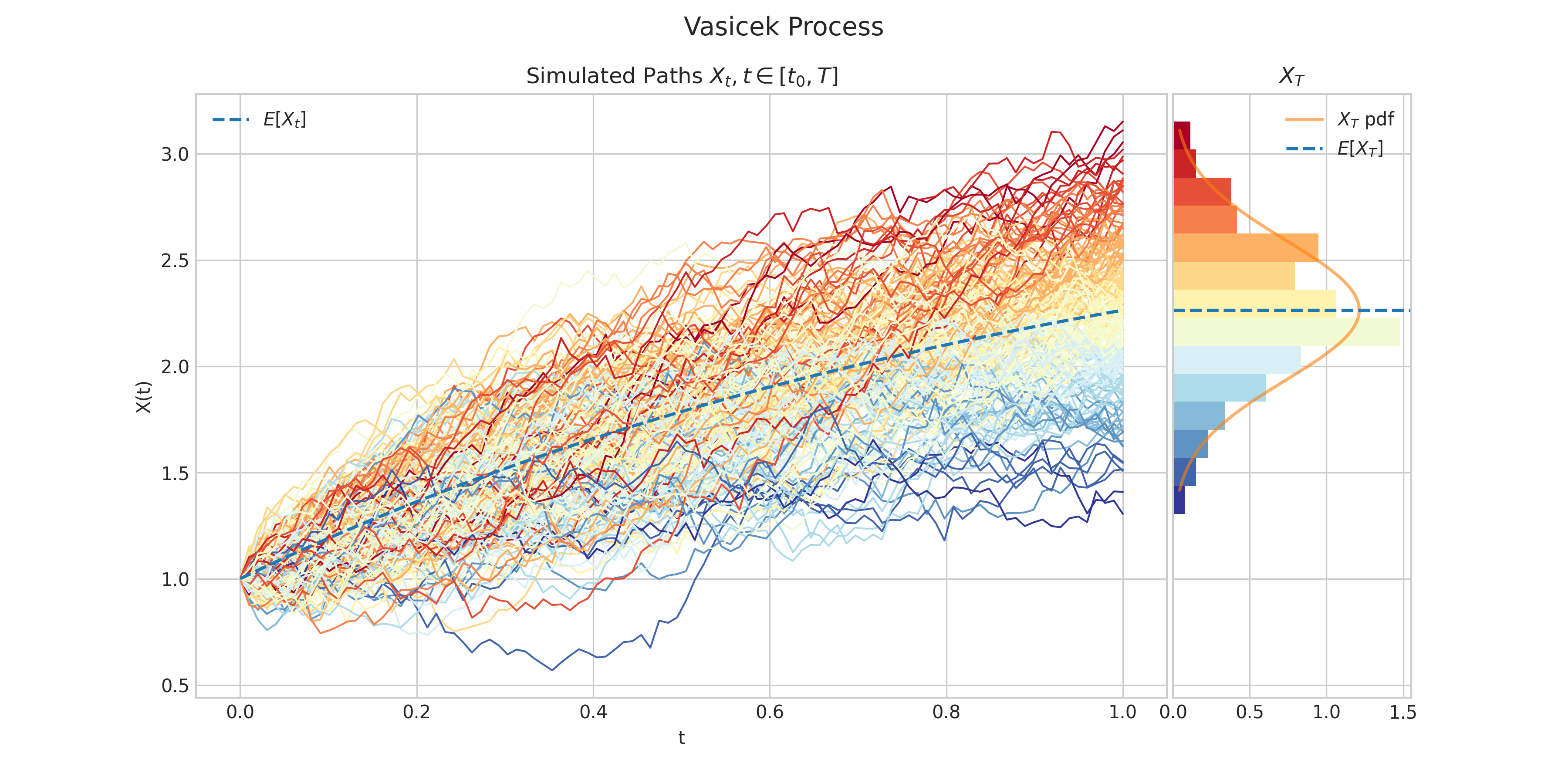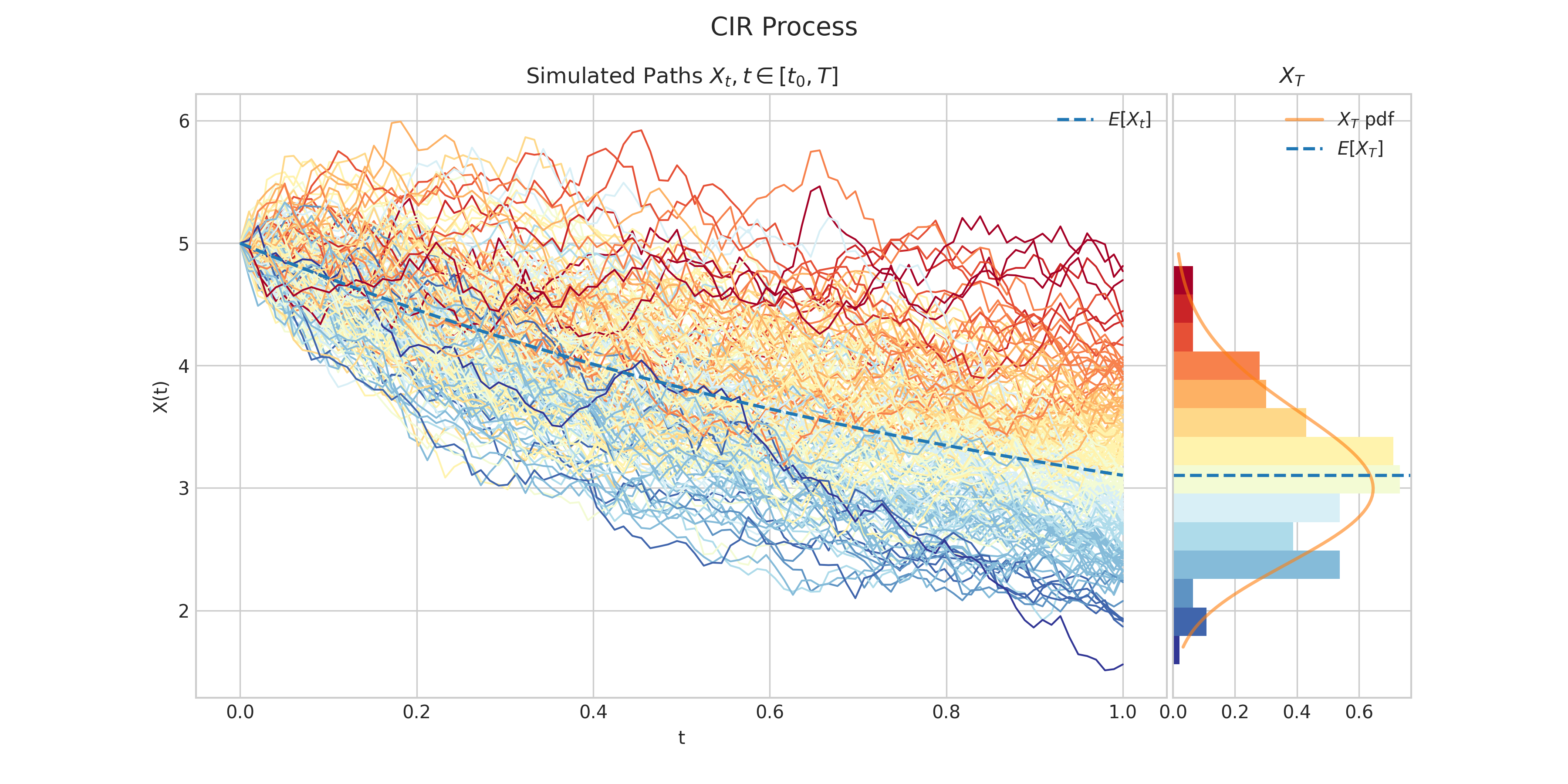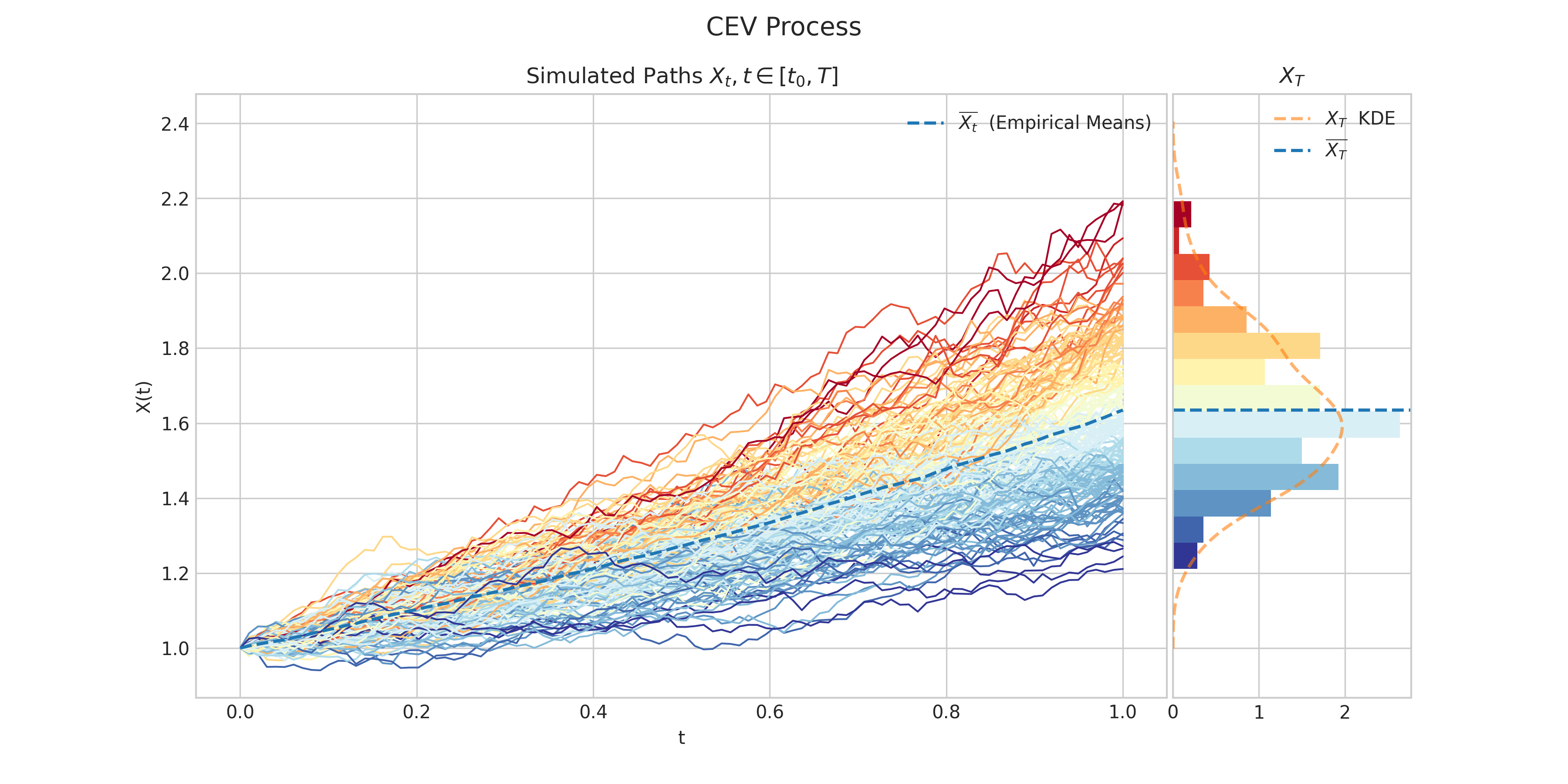# EM Processes#

The aleatory.processes module also provides functionality for continuous-time stochastic processes which cannot be expressed in analytical form. These processes are simulated via the Euler-Maruyama method.

class aleatory.processes.OUProcess(theta=1.0, sigma=0.5, initial=1.0, T=1.0, rng=None)[source]#

Ornstein–Uhlenbeck ProcessAn Ornstein–Uhlenbeck process $$X = \{X : t \geq 0\}$$ is characterised by the following Stochastic Differential Equation

$dX_t = -\theta X_t dt + \sigma X_t dW_t, \ \ \ \ \forall t\in (0,T],$

with initial condition $$X_0 = x_0$$, where

• $$\theta$$ is the speed of reversion

• $$\sigma>0$$ is the instantaneous volatility

• $$W_t$$ is a standard Brownian Motion.

Each $$X_t$$ follows a normal distribution.

Parameters:
• theta (float) – the parameter $$\theta$$ in the above SDE

• sigma (float) – the parameter $$\sigma>0$$ in the above SDE

• initial (float) – the initial condition $$x_0$$ in the above SDE

• T (float) – the right hand endpoint of the time interval $$[0,T]$$ for the process

• rng (numpy.random.Generator) – a custom random number generator

property T#

End time of the process.

draw(n, N, marginal=True, envelope=False, title=None, **fig_kw)#

Simulates and plots paths/trajectories from the instanced stochastic process. Visualisation shows - times versus process values as lines - the expectation of the process across time - histogram showing the empirical marginal distribution $$X_T$$ - probability density function of the marginal distribution $$X_T$$ - envelope of confidence intervals

Parameters:
• n – number of steps in each path

• N – number of paths to simulate

• marginal – bool, default: True

• envelope – bool, default: False

Returns:

plot(n, N, title=None, **fig_kw)#

Simulates and plots paths/trajectories from the instanced stochastic process. Simple plot of times versus process values as lines and/or markers.

Parameters:
• n – number of steps in each path

• N – number of paths to simulate

• title – string to customise plot title

Returns:

simulate(n, N)#

Simulate paths/trajectories from the instanced stochastic process.

Parameters:
• n – number of steps in each path

• N – number of paths to simulate

Returns:

list with N paths (each one is a np.array of size n)

class aleatory.processes.Vasicek(theta=1.0, mu=3.0, sigma=0.5, initial=1.0, T=1.0, rng=None)[source]#

Vasicek ProcessA Vasicek process $$X = \{X : t \geq 0\}$$ is characterised by the following Stochastic Differential Equation

$dX_t = \theta(\mu - X_t) dt + \sigma X_t dW_t, \ \ \ \ \forall t\in (0,T],$

with initial condition $$X_0 = x_0$$, where

• $$\theta$$ is the speed of reversion

• $$\mu$$ is the long term mean value.

• $$\sigma>0$$ is the instantaneous volatility

• $$W_t$$ is a standard Brownian Motion.

Each $$X_t$$ follows a normal distribution.

Parameters:
• theta (float) – the parameter $$\theta$$ in the above SDE

• mu (float) – the parameter $$\mu$$ in the above SDE

• sigma (float) – the parameter $$\sigma>0$$ in the above SDE

• initial (float) – the initial condition $$x_0$$ in the above SDE

• T (float) – the right hand endpoint of the time interval $$[0,T]$$ for the process

• rng (numpy.random.Generator) – a custom random number generator

property T#

End time of the process.

draw(n, N, marginal=True, envelope=False, title=None, **fig_kw)#

Simulates and plots paths/trajectories from the instanced stochastic process. Visualisation shows - times versus process values as lines - the expectation of the process across time - histogram showing the empirical marginal distribution $$X_T$$ - probability density function of the marginal distribution $$X_T$$ - envelope of confidence intervals

Parameters:
• n – number of steps in each path

• N – number of paths to simulate

• marginal – bool, default: True

• envelope – bool, default: False

Returns:

plot(n, N, title=None, **fig_kw)#

Simulates and plots paths/trajectories from the instanced stochastic process. Simple plot of times versus process values as lines and/or markers.

Parameters:
• n – number of steps in each path

• N – number of paths to simulate

• title – string to customise plot title

Returns:

simulate(n, N)#

Simulate paths/trajectories from the instanced stochastic process.

Parameters:
• n – number of steps in each path

• N – number of paths to simulate

Returns:

list with N paths (each one is a np.array of size n)

class aleatory.processes.CIRProcess(theta=1.0, mu=2.0, sigma=0.5, initial=5.0, T=1.0, rng=None)[source]#

Cox–Ingersoll–Ross ProcessA Cox–Ingersoll–Ross process $$X = \{X : t \geq 0\}$$ is characterised by the following Stochastic Differential Equation

$dX_t = \theta(\mu - X_t) dt + \sigma \sqrt{X_t} dW_t, \ \ \ \ \forall t\in (0,T],$

with initial condition $$X_0 = x_0$$, where

• $$\theta$$ is the rate of mean reversion

• $$\mu$$ is the long term mean value.

• $$\sigma>0$$ is the instantaneous volatility

• $$W_t$$ is a standard Brownian Motion.

Each $$X_t$$ follows a non-central chi-square distribution.

Parameters:
• theta (float) – the parameter $$\theta$$ in the above SDE

• mu (float) – the parameter $$\mu$$ in the above SDE

• sigma (float) – the parameter $$\sigma>0$$ in the above SDE

• initial (float) – the initial condition $$x_0$$ in the above SDE

• T (float) – the right hand endpoint of the time interval $$[0,T]$$ for the process

• rng (numpy.random.Generator) – a custom random number generator

property T#

End time of the process.

draw(n, N, marginal=True, envelope=False, title=None, **fig_kw)#

Simulates and plots paths/trajectories from the instanced stochastic process. Visualisation shows - times versus process values as lines - the expectation of the process across time - histogram showing the empirical marginal distribution $$X_T$$ - probability density function of the marginal distribution $$X_T$$ - envelope of confidence intervals

Parameters:
• n – number of steps in each path

• N – number of paths to simulate

• marginal – bool, default: True

• envelope – bool, default: False

Returns:

plot(n, N, title=None, **fig_kw)#

Simulates and plots paths/trajectories from the instanced stochastic process. Simple plot of times versus process values as lines and/or markers.

Parameters:
• n – number of steps in each path

• N – number of paths to simulate

• title – string to customise plot title

Returns:

simulate(n, N)#

Simulate paths/trajectories from the instanced stochastic process.

Parameters:
• n – number of steps in each path

• N – number of paths to simulate

Returns:

list with N paths (each one is a np.array of size n)

class aleatory.processes.CEVProcess(gamma=1.5, mu=0.5, sigma=0.1, initial=1.0, T=1.0, rng=None)[source]#

CEV or constant elasticity of variance processA CEV process $$X = \{X : t \geq 0\}$$ is characterised by the following Stochastic Differential Equation

$dX_t = \mu X_t dt + \sigma X_t^{\gamma} dW_t, \ \ \ \ \forall t\in (0,T],$

with initial condition $$X_0 = x_0$$, where

• $$\mu$$ is the drift

• $$\sigma>0$$ is the scale of the volatility

• $$\gamma\geq 0$$ is the elasticity term

• $$W_t$$ is a standard Brownian Motion.

Parameters:
• mu (float) – the parameter $$\mu$$ in the above SDE

• sigma (float) – the parameter $$\sigma>0$$ in the above SDE

• gamma (float) – the parameter $$\gamma$$ in the above SDE

• initial (float) – the initial condition $$x_0$$ in the above SDE

• T (float) – the right hand endpoint of the time interval $$[0,T]$$ for the process

• rng (numpy.random.Generator) – a custom random number generator

property T#

End time of the process.

draw(n, N, marginal=True, envelope=False, title=None, **fig_kw)[source]#

Simulates and plots paths/trajectories from the instanced stochastic process. Visualisation shows - times versus process values as lines - the expectation of the process across time - histogram showing the empirical marginal distribution $$X_T$$ - probability density function of the marginal distribution $$X_T$$ - envelope of confidence intervals

Parameters:
• n – number of steps in each path

• N – number of paths to simulate

• marginal – bool, default: True

• envelope – bool, default: False

Returns:

plot(n, N, title=None, **fig_kw)#

Simulates and plots paths/trajectories from the instanced stochastic process. Simple plot of times versus process values as lines and/or markers.

Parameters:
• n – number of steps in each path

• N – number of paths to simulate

• title – string to customise plot title

Returns:

simulate(n, N)#

Simulate paths/trajectories from the instanced stochastic process.

Parameters:
• n – number of steps in each path

• N – number of paths to simulate

Returns:

list with N paths (each one is a np.array of size n)